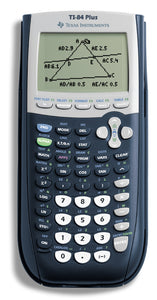# TI-84 Plus Calculator (Used)

• \$70.00
Unit price per
Shipping calculated at checkout.

Expanded graphing technology performance comes preloaded with more than a dozen applications, including functionality that encourages exploration of interactive geometry, inequality graphing and real-world data collection and analysis.

Product Type:   Graphing Calculator

### Technical Information

Operations/Functionality:
• Real and complex numbers calculated to 14-digit accuracy and displayed with 10 digits plus a 2-digit exponent
• Graphs 10 rectangular functions, 6 parametric expressions, 6 polar expressions and 3 recursively-defined sequences
• Up to 10 graphing functions defined, saved, graphed and analyzed at one time
• Sequence graphing mode shows time series plot, cobweb/ stair-step plot and phase plots
• User-defined list names. Lists store up to 999 elements
• 14 interactive zoom features
• Function evaluation table shows numeric evaluation of functions in table format
• Interactive analysis of function values, roots, maximums, minimums, integrals and derivatives
• 7 different graph styles for differentiating the look of each graph drawn
• Horizontal and vertical split- screen options
• Matrix operations including inverse, determinant, transpose, augment, reduced row echelon form and elementary row operations. Convert matrices to lists and vice-versa
• List-based one- and two-variable statistical analysis, including logistic, sinusoidal, median-median, linear, logarithmic, exponential, power, quadratic polynomial, cubic polynomial, and quadratic polynomial regression models
• 3 statistical plot definitions for scatter plots, xy-line plots, histograms, regular and modified box-and-whisker plots, and normal probability plots
• Advanced statistics features including 10 hypothesis testing functions, 7 confidence interval functions and one-way analysis of variance
• 15 probability distribution functions including Normal, Student-t, Chi-square, Binomial and Poisson
• Business functions including Time-Value-of-Money (TVM), cash flows, and amortization. Full screen interactive editor for solving TVM problems
• Interactive equation solver editor for solving for different variables in an equation.
• Alphabetical CATALOG of all TI calculator operations in one menu
• All TI-83 Plus graphing calculators are fully compatible with TI-84 Plus family calculators